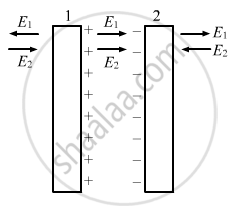Department of Pre-University Education, Karnataka course PUC Karnataka Science Class 12
Share

# Charged Particle with a Charge of −2⋅0 × 10−6 C is Placed Close to a Non-conducting Plate with a Surface Charge Density of 4 ⋅ 0 × 10 − 6 C M − 2 . - Physics

ConceptElectric Field Introduction of Electric Field

#### Question

A charged particle with a charge of −2⋅0 × 10−6 C is placed close to a non-conducting plate with a surface charge density of 4.0 × 10-6Cm0-2. Find the force of attraction between the particle and the plate.

#### Solution

The electric field due to a conducting thin sheet,

"E" = sigma/( 2 ∈ _0)

The magnitude of attractive force between the particle and the plate,

F =qE

"F" = (q xx sigma)/(2∈_0)

"F" = ((2.0 xx 10^-6 ) xx ( 4.0 xx 10^-6))/(2 xx (8.55 xx 10^-12))

F = 0.45 NIs there an error in this question or solution?

#### APPEARS IN

Solution Charged Particle with a Charge of −2⋅0 × 10−6 C is Placed Close to a Non-conducting Plate with a Surface Charge Density of 4 ⋅ 0 × 10 − 6 C M − 2 . Concept: Electric Field - Introduction of Electric Field.
S